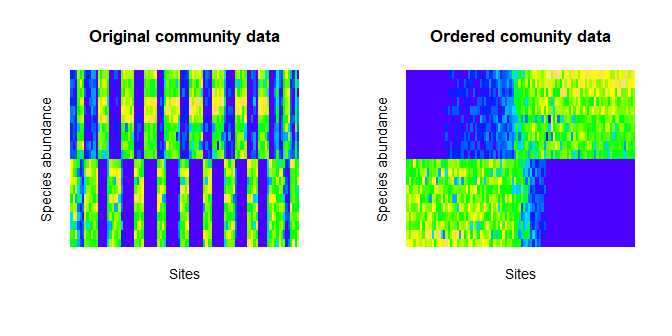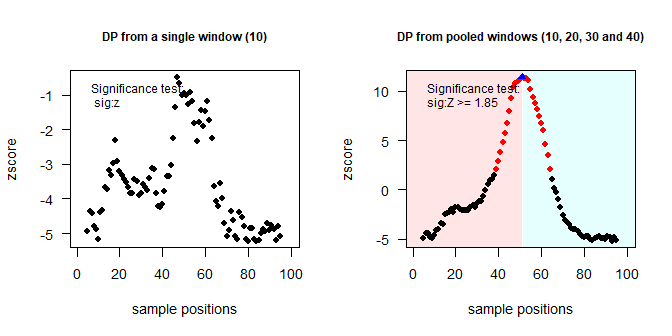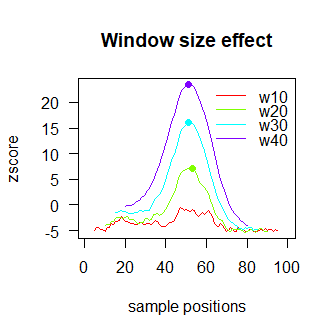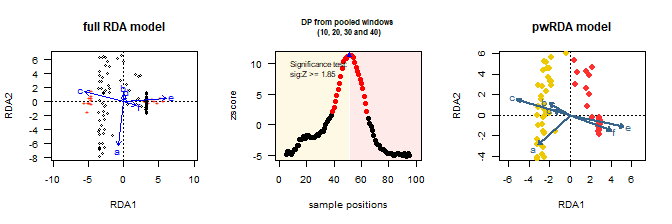segRDA vignette

June 22, 2018

segRDA is designed to model non-continuous linear responses of ecological communities to environmental data. This tutorial assumes familiarity both with R and with community ordination. The package segRDA is available on CRAN or the latest development version can be installed from GitHub using the package devtools : https://github.com/DaniloCVieira/segRDA.

# to install the package via CRAN
install.packages("segRDA")

# to install the package via GitHub
devtools::install_github("DaniloCVieira/segRDA")

The modeling process with segRDA is straightforward through three steps: (1) data ordering (function OrdData), (2) split-moving-window analysis (function SMW) and (3) piecewise redundancy analysis (function pwRDA). segRDA provides simualted and empirical datasets to facilitate reproducible examples. Each of these dataset is a list composed of two matrices: envi and comm. Through the following example code we will use the dataset sim1 from the package. At the end of this document, we present the key running procedures for the empirical dataset nema.

data(sim1) ##Simulated data
x<-sim1$envi ## matrix of explanatory variables y<-sim1$comm ## matrix of response variables

Data ordering

Both the SMW and pwRDA analyses depend on ordered datasets. The ordering can be user-defined or generated using OrdData function. The latter is a wrapper for (1) performing a redundancy analysis using the rda function from vegan package and (2) ordering both response and explanatory matrices using one of the axes of the RDA model. Defaults to use the first RDA axis. The function also allows the user to transform community data prior to RDA analysis. The transformation is specified by the argument method, which is further passed to the decostand function from vegan. Additional arguments from rda and decostand can be passed directly to the OrdData function.

sim1o<-OrdData(x=x,y=y, axis=1, method="hellinger")

OrdData returns an object of class ord, which is a list consisting of:

• ..$xo: the ordered explanatory matrix; • ..$yo: the ordered community matrix (untransformed);
• ..$x: the original explanatory matrix; • ..$y: the original community matrix;
xo<-sim1o$xo ## ordered explanatory matrix. yo<-sim1o$yo ## ordered community matrix (untransformed).

The resulting ordered community can be represented by a species-site interaction matrix using the function image, which is available from the stats package of R.

par(mfrow = c(1, 2), mgp = c(1, 1, 0), cex = 0.9)
image(y, main = "Original community data", col = topo.colors(100), axes = F,
xlab = "Sites", ylab = "Species abundance")
image(yo, main = "Ordered comunity data", col = topo.colors(100), axes = F,
xlab = "Sites", ylab = "Species abundance")Split-moving window analysis

A brief overview of the SMW analysis.

The application of SMW applied to a species matrix allows consists of (1) placing a window of even-numbered size at the beginning of the data series, (2) splitting the window into two equal halves, (3) calculating the community centroids within each half, (4) computing a dissimilarity metric between the two halves, (5) shifting window one position along the series, and (6) repeating the procedure till the end of the data series (Cornelius & Reynolds 1991). The significance of the dissimilarity values within each window is tested through multi-response permutations and includes two types of procedures: the “random-plot” and “random-shift”. The first randomizes sites along the series while preserving species composition and abundance structure of the sites. The second randomizes patterns of each species relative to each other while preserving the spatial structure of the species abundance. Therefore, choosing between random plot and random shift relies on considering sites or species as a fixed aspect of the null distribution. Both procedures include computing an expected mean dissimilarity (DS) and standard deviation (SD) for each window midpoint. Confidence limits have been suggested as one or two SD above DS or estimated from one-tailed 95% confidence intervals (Erdos et al. 2014).

The choice of window size affects the results of the SMW analysis. A pooled profile by averaging together dissimilarities from different window sizes reduces the scale-effect. In this case, dissimilarity profiles of the mean Z-score (standardized values of dissimilarity) are used to detect the community breakpoints.

In plot randomization, Z-scores increase at each window midpoint together with an increase in window width, but the general shape of the dissimilarity profiles remains unchanged. In contrast, the random-shift method minimizes the scale dependence of Z-scores and makes a clear distinction between non-significant and significant discontinuities (Körmöczi et al. 2016).

The function SMW

The package segRDA implements the SMW analysis through the function SMW which requires the following arguments:

• yo: the ordered community;
• ws: either a single window size or a vector of several windows sizes;
• dist: the distance metric (all distances metrics available in vegan::vegdist);
• rand: the randomization type ("shift" or "plot");
• n.rand: the number of randomizations;

The default settings are dist="bray", rand="shift" and n.rand=99. The running time of the analysis will depend on the number of window sizes (length(ws)) multiplied by the number of randomizations n.rand. In the following examples, we settled a low number of randomizations to speed up the analyses with the remaining arguments left at their default values.

SMW returns invisibly an smw object, which is a two-level list object describing the SMW results for each window w analyzed. Each of the w slots is a list containing the following results:

• ..$dp: The raw dissimilarity profile table (DP): a data frame giving the positions, labels, values of dissimilarity and z-scores for each sample; • ..$rdp: data frame containing the randomized DP;
• ..$md: mean dissimilarity of the randomized DP; • ..$sd: standard deviation for each sample position;
• ..$oem: overall expected mean dissimilarity; • ..$osd: average standard deviation for the dissimilarities;
• ..$params: list with input arguments. Methods for extracting and plotting the SMW results extract The function extract allows the user to access the results cointained in a smw object. The argument index specifies which of the results described above will be extracted. By default, extract returns an object of class dp: a DP table containing significant discontinuities and suggested breakpoints. Extracting a dp object implements the following auxiliary arguments: • sig: defines the statistical test used to detect significant discontinuities. The statistical tests available are "z","sd","sd2", and "tail1"; • z: sets the critical value valor for z-scores when sig="z"; • BPs: defines if the breakpoints should be chosen as those site positions corresponding to the maximum dissimilarity in a sequence of significant dissimilarity values (BPs="max") or as those site positions corresponding to the median position of the sequence (BPs="median"). If NULL the breakpoints are not computed; • seq.sig: specifies the length of a sequence of significant dissimilarity values that will be considered in defining the community breakpoints. The default settings are index="dp", sig="z", z="1.85", BPs="max" and seq.sig=3. The returned dp object has its own generic print method, print.dp. When the smw object handles multiple window sizes (hereafter referred to as pooled smw objects), the extracted DP table will contain the z-scores averaged over the set of window sizes. A specific DP can be extracted from pooled objects by specifying a target window size (argument w). The length of consecutive, significant dissimilarity values (argument seq.sig) is used as criteria for defining breakpoints. The function will display a warning if seq.sig exceeds the maximum threshold: The function bp is an auxiliary tool for displaying the breakpoints positions in objects of class dp (if present): Note that all other results contained in smw objects (i.e. rdp, md, sd, oem, osd and params ) comprise results of single window sizes. Thus, the argument w must be specified to access them from pooled objects. plot The smw object has a generic plot method, plot.smw. This command is a convenience wrapper for accessing and viewing the results cointained in a smw object. Auxiliary arguments from extract (i.e. sig, z, BPs and seq.sig) can be passed to plot.smw. The function returns invisibly a DP table (object class dp) and plots the location of the window midpoints vs. dissimilarity values. By default, significant dissimilarity values are given in “red” and the breakpoints in “blue”. The background area of the graph can be coloured according to the breakpoints location (argument bg). These colors are controled by the arguments bg and bg_alpha. The colors generated for each sample can be acessed through the command bgDP(dp) (where dp is an object of class dp). As we shall see later, this vector may be useful in the simultaneous visualization of the results the SMW and pwRDA. For the complete list of graphical parameters, please go to help(plot.smw).A second type of plot is available for pooled smw objects: the window size effect. When the smw object is pooled and the argument w.effect is TRUE, the plot method drawns together the profiles obtained from the different window sizes.Piecewise redundancy analysis (pwRDA) Once the breakpoints have been defined and their significance tested, the pwRDA can be implemented. It is done with the function pwRDA. The function has three arguments: x explanatory variables, y response variables and BPs the positions of the breakpoints. The function displays a message with the main result of the analysis: pw.sim<-pwRDA(x.ord=xo,y.ord=yo, BPs=bp(pool_dp)) The function returns an invisible list with following descriptors: • ..$summ: a vector containing the summarized statistics.
• ..$rda.0 and ..$rda.pw: the respectives cca objects from the full and “pw”" RDA models. These objects can be used in all functions that applies to vegan::cca.object. More details for accessing and plotting a cca.object are in help(cca.object) and help(plot.cca), respectivaly.

The command bgDP applied to the object returned by the fuction plot.smw returns the bg colors for each sample. It can be used for a simultaneous view of the results of the SMW and pwRDA analyses.

head(pw.sim$summ) ## Statistic P.value ## FULL 0.6446842 0.0010000000 ## PW 0.8240577 0.0000045212 ## F 3.1083641 0.0082726695 par(mfrow = c(1, 3), cex = 0.65) # plotting the full rda model: plot(pw.sim$rda.0, main = "full RDA model", las = 1)
# plotting the DP profile and saving the output in an new object
dp <- plot(pool, main = "DP from pooled windows \n (10, 20, 30 and 40)",
bg = c("gold2", "firebrick1"), cex.main = 0.8)

# plotting the pwRDA colored according to the breakpoints:
plot(pw.sim$rda.pw, type = "n", scaling = 3, main = "pwRDA model") points(pw.sim$rda.pw, pch = 16, col = bgDP(dp), cex = 1.2)
text(pw.sim\$rda.pw, display = "bp", pch = 16, col = "steelblue4", lwd = 2)Literature

1. Burrough, P.A. (1986). Principles of Geographical Information Systems for Land Resources Assessment. Journal of Quaternary Sciencee, 193.

2. Cornelius, J.M. & Reynolds, J.F. (1991). On Determining the Statistical Significance of Discontinuities with Ordered Ecological Data. Ecology, 72, 2057–2070.

3. Erdos, L., Bátori, Z., Tölgyesi, C.S. & Körmöczi, L. (2014). The moving split window (MSW) analysis in vegetation science - An overview. Applied Ecology and Environmental Research, 12, 787–805.

4. Körmöczi, L., Bátori, Z., Erdős, L., Tölgyesi, C., Zalatnai, M. & Varró, C. (2016). The role of randomization tests in vegetation boundary detection with moving split-window analysis. Journal of Vegetation Science, 27, 1288–1296.

5. Oksanen, J., Blanchet, F.G., Friendly, M., Kindt, R., Legendre, P., McGlinn, D., Minchin, P.R., O’Hara, R.B., Simpson, G.L., Solymos, P., Stevens, M.H.H., Szoecs, E. & Wagner, H. (2017). vegan: Community Ecology Package.